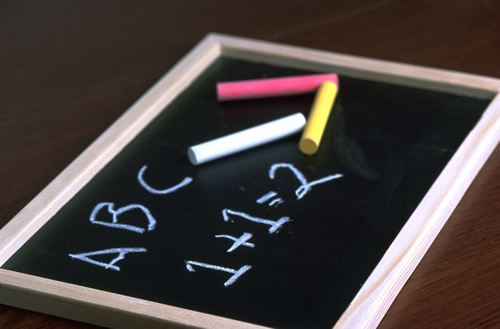•Doubles Facts

1 + 1 = 2
2 + 2 = 4
3 + 3 = 6
4 + 4 = 8
5 + 5 = 10
6 + 6 = 12
7 + 7 = 14
8 + 8 = 16
9 + 9 = 18
10 + 10 = 20

Almost Doubles

1 + 2 = 3
2 + 3 = 5
3 + 4 = 7
4 + 5 = 9
5 + 6 = 11
6 + 7 = 13
7 + 8 = 15
8 + 9 = 17

Ten Friends

1 + 9 = 10
2 + 8 = 10
3 + 7 = 10
4 + 6 = 10
5 + 5 = 10
6 + 4 = 10
7 + 3 = 10
8 + 2 = 10
9 + 1 = 10

10 More

1 + 10 = 11
2 + 10 = 12
3 + 10 = 13
4 + 10 = 14
5 + 10 = 15
6 + 10 = 16
7 + 10 = 17
8 + 10 = 18
9 + 10 = 19
10 + 10 = 20

9 More

1 + 9 = 10
2 + 9 = 11
3 + 9 = 12
4 + 9 = 13
5 + 9 = 14
6 + 9 = 15
7 + 9 = 16
8 + 9 = 17
9 + 9 = 18
10 + 9 = 19